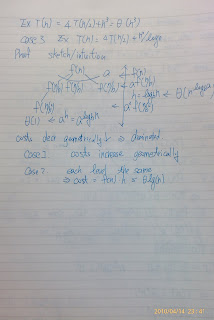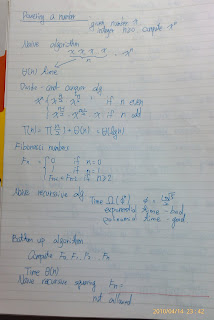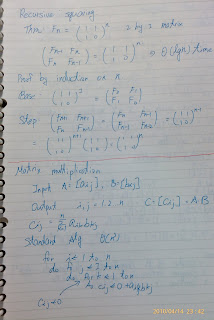## Wednesday, April 14, 2010

### Algorithm lecture 4

divide and conquer有三部曲，顧名思義 divide conquer 還有一個combine。

Divide -> partition array into 2 subarrays around pivot x.

Conquer -> recursively sort 2 subarrays
Combine -> Trivial

1.substitution method
step1:
Guess the form of the solution.
step2:Use mathematical induction to find the constants and show that the solution works.

2.recursion-tree

3.master method

T(n) = aT(n/b) + f(n)